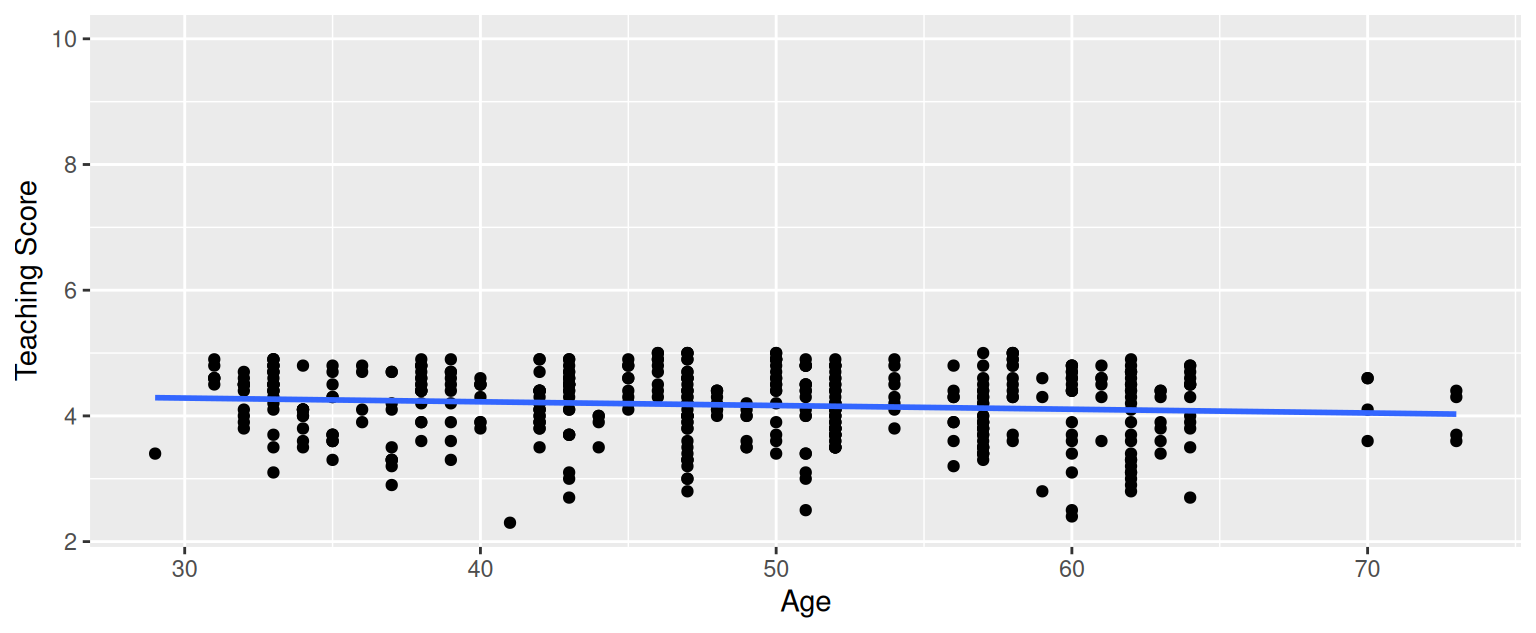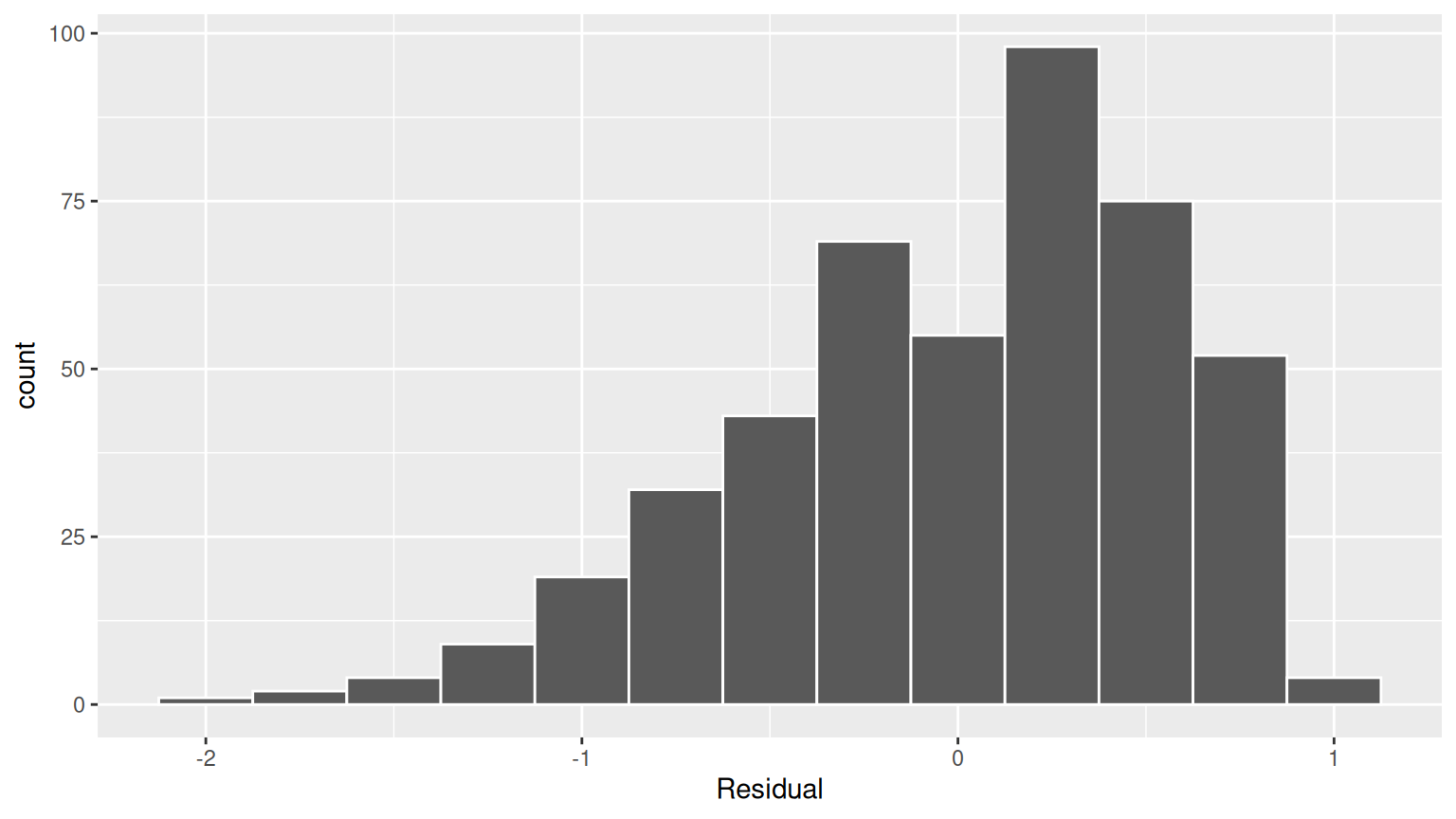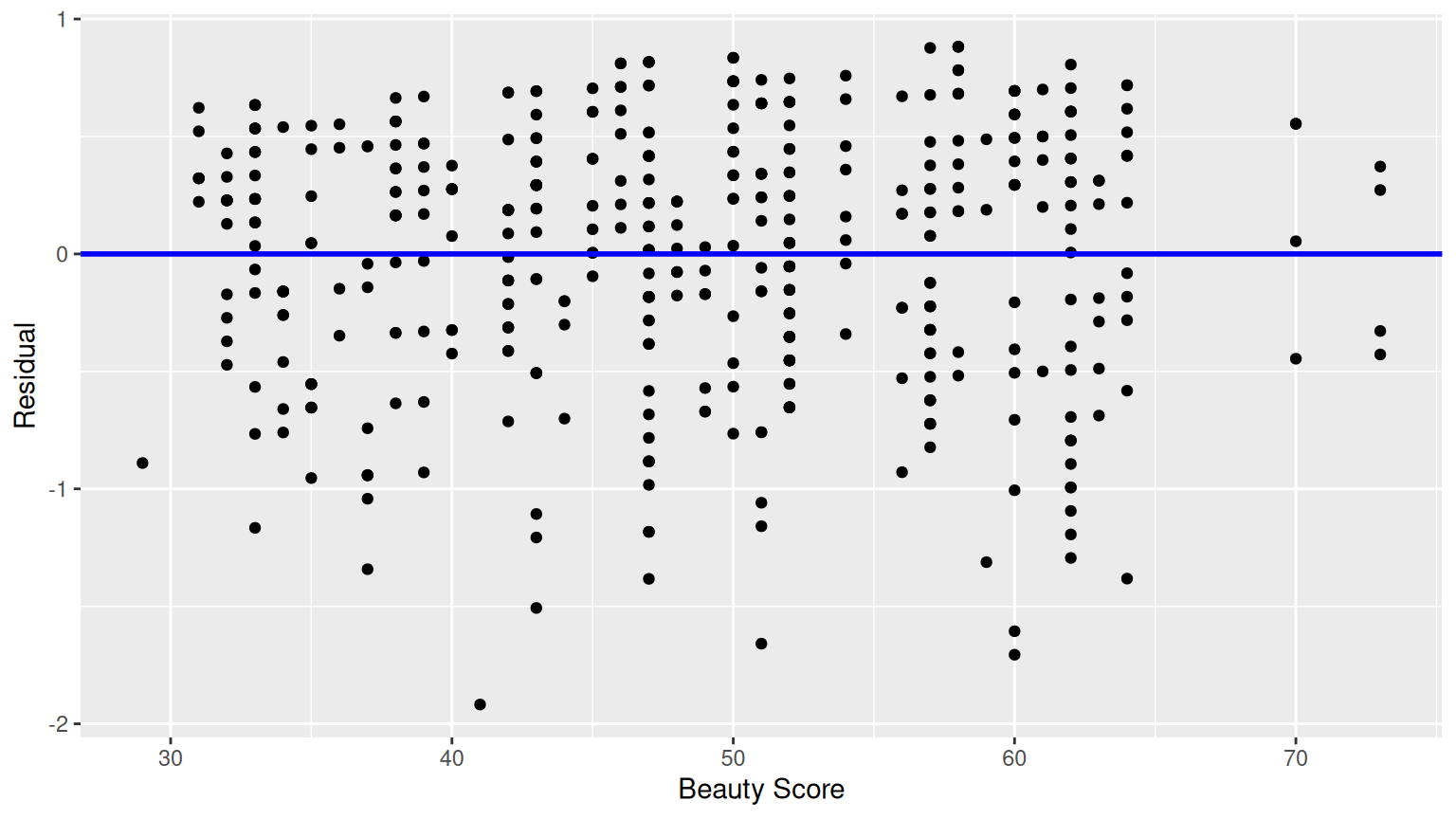## D.10 Chapter 10 Solutions

library(tidyverse)
library(moderndive)
library(infer)

(LC10.1) Continuing with our regression using age as the explanatory variable and teaching score as the outcome variable.

• Use the get_regression_points() function to get the observed values, fitted values, and residuals for all 463 instructors.
evals_ch5 <- evals %>%
select(ID, score, bty_avg, age)

# Fit regression model:
score_age_model <- lm(score ~ age, data = evals_ch5)
# Get regression points:
regression_points <- get_regression_points(score_age_model)
regression_points
# A tibble: 463 x 5
ID score   age score_hat residual
<int> <dbl> <int>     <dbl>    <dbl>
1     1 4.7      36     4.248  0.452
2     2 4.100    36     4.248 -0.148
3     3 3.9      36     4.248 -0.34800
4     4 4.8      36     4.248  0.552
5     5 4.600    59     4.112  0.488
6     6 4.3      59     4.112  0.188
7     7 2.8      59     4.112 -1.312
8     8 4.100    51     4.159 -0.059
9     9 3.4      51     4.159 -0.759
10    10 4.5      40     4.224  0.276
# … with 453 more rows
• Perform a residual analysis and look for any systematic patterns in the residuals. Ideally, there should be little to no pattern but comment on what you find here.

The first condition is that the relationship between the outcome variable $$y$$ and the explanatory variable $$x$$ must be Linear.FIGURE D.3: Example of a clearly non-linear relationship.

The relationship between score and age does not seem to be linear.

The second condition is that the residuals must be Independent. In other words, the different observations in our data must be independent of one another. As explained in 10.3.3, “we say there exists dependence between observations”.

The third condition is that the residuals should follow a Normal distribution.FIGURE D.4: Histogram of residuals.

The fourth and final condition is that the residuals should exhibit Equal variance across all values of the explanatory variable $$x$$. In other words, the value and spread of the residuals should not depend on the value of the explanatory variable $$x$$.FIGURE D.5: Plot of residuals over beauty score.

This plot seems to fit equality of variance.

(LC10.2) Repeat the inference but this time for the correlation coefficient instead of the slope. Note the implementation of stat = "correlation" in the calculate() function of the infer package.

bootstrap_distn_slope <- evals_ch5 %>%
specify(formula = score ~ bty_avg) %>%
generate(reps = 1000, type = "bootstrap") %>%
calculate(stat = "correlation")

if (!file.exists("rds/bootstrap_distn_slope.rds")) {
set.seed(76)
bootstrap_distn_slope <- evals %>%
specify(score ~ bty_avg) %>%
generate(reps = 1000, type = "bootstrap") %>%
calculate(stat = "slope")
saveRDS(
object = bootstrap_distn_slope,
"rds/bootstrap_distn_slope.rds"
)
} else {
observed_slope